### Home > CALC > Chapter Ch7 > Lesson 7.1.1 > Problem7-6

7-6.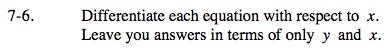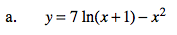$\text{The derivative of ln}(x)=\frac{1}{x}.$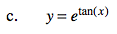Chain Rule.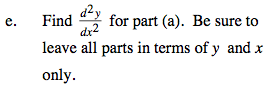$\frac{d^{2}y}{dx^{2}}=\frac{d}{dx}\text{(answer to part (a)) }.$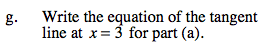The equation for the tangent line is yy(3) = y'(3)(x − 3).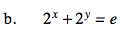Implicit Differentiation.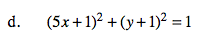$\text{Implicit differentiation. Solve for }\frac{dy}{dx}.$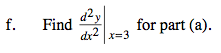Translation: Evaluate the 2nd-derivative (that you found in part (e)) at x = 3.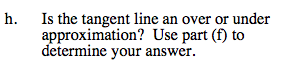Is the graph concave up or concave down?